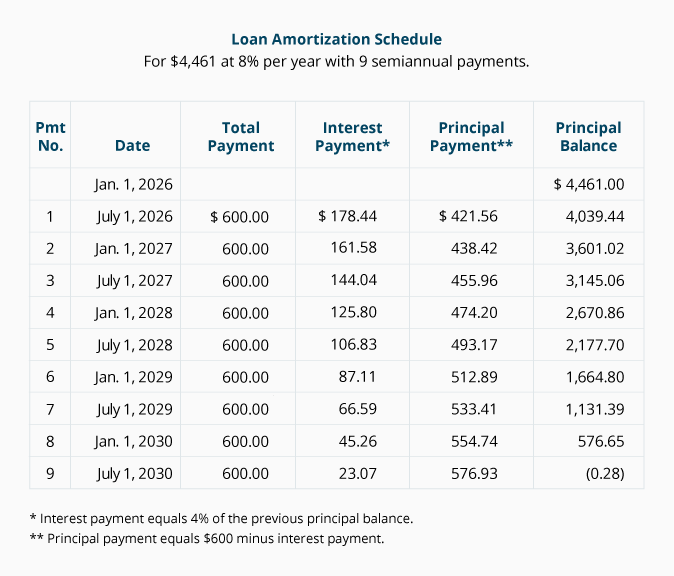# Calculating the Length of an Ordinary Annuity (n)

We can use present value calculations to determine the number of periods (or payments) in an ordinary annuity if we know the other components: present value, interest rate, and the amount of each recurring payment. Exercises 7 and 8 below demonstrate how to solve for the number of periods (or payments).

### Exercise #7

Janine has an investment account with a present value of \$5,053. The account earns interest at 6% per year compounded annually. She intends to withdraw \$500 at the end of each year. Assume that the present time (period 0) is January 1, 2023 and the first withdrawal will take place on December 31, 2023. How many withdrawals can Janine make before her account balance shrinks to \$0?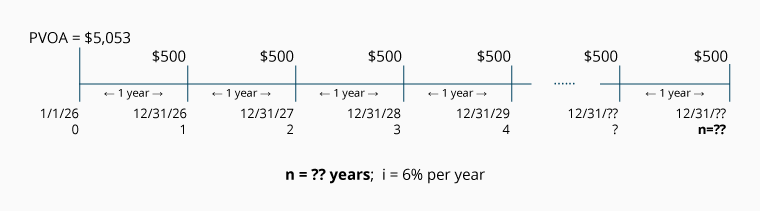Calculation of Exercise #7 using the PVOA Table

Solving for n (the number of periods/payments in an ordinary annuity) is done with the following equation: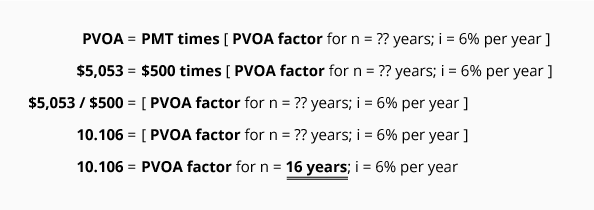Let's review this calculation. We insert into the equation the components that we know: the present value, the interest rate, and the recurring payment amount. In line four, we calculate our factor to be 10.106. We now know both the PVOA factor (10.106) and the interest rate (6%). We go to the PVOA Table and look down the 6% interest column until we come to the factor 10.106. Tracking across the row, we see that at this point, n = 16. Since the periods in question are annual periods, the answer is 16 years.

Here is the proof of this calculation:

Investment Account Activity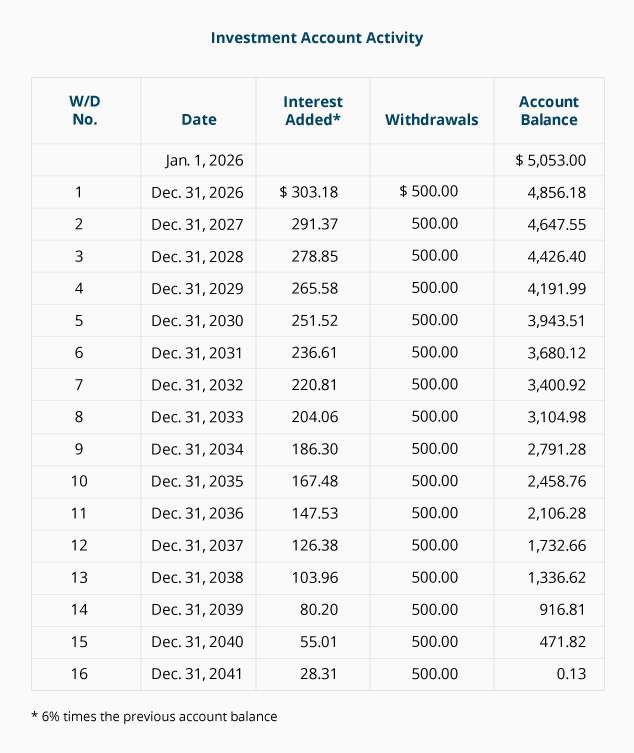### Exercise #8

Jeremy borrows \$4,461 at an interest rate of 8% per year, compounded semiannually on each July 1 and January 1. He plans to make a \$600 loan payment at the end of each semiannual period. Assuming that the present time (period 0) is January 1, 2023 and the first loan payment will take place on July 1, 2023, when will the loan balance be \$0?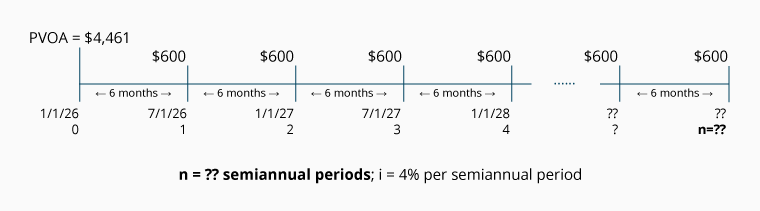Calculation of Exercise #8 using the PVOA Table

The number of semiannual periods/payments in the ordinary annuity can be computed with the PVOA equation: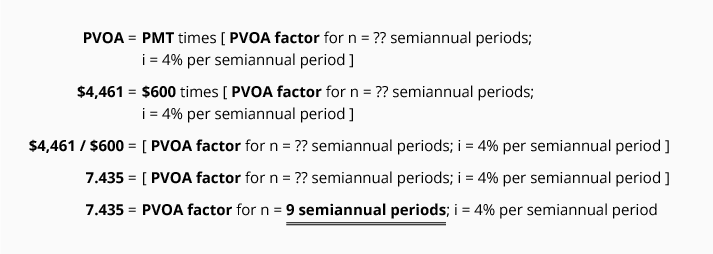Let's review this calculation. We insert into the equation the components that we know: the present value, the interest rate, and the recurring payment amount. In line four, we calculate our factor to be 7.435. We now know both the PVOA factor (7.435) and the interest rate (4%). We go to the PVOA Table and look down the 4% interest column until we come to the factor 7.435. Tracking across the row, we see that at this point, n = 9. Since the periods in question are semiannual periods, the answer is 9 semiannual periods.

The question we were asked is "when will the loan balance be \$0?" The answer is July 1, 2027. Here is the proof of this calculation:

Loan Amortization Schedule
(For \$4,461 at 8% per year with 9 semiannual payments.)